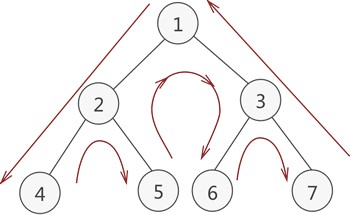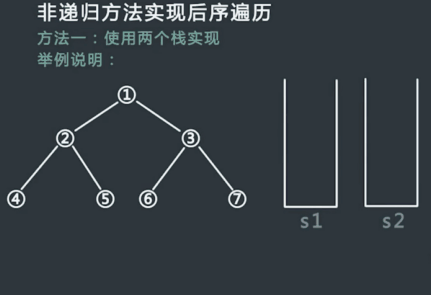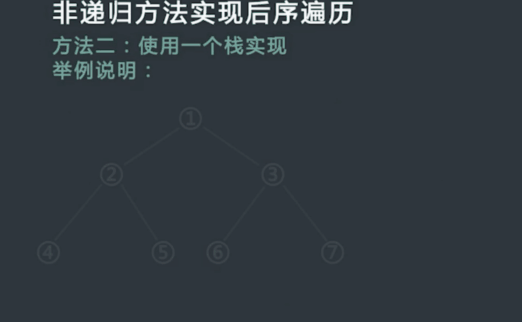## 二叉树节点定义

 ``````1 2 3 4 5 6 `````` ``````struct TreeNode { int val; TreeNode *left; TreeNode *right; TreeNode(int x) : val(x), left(NULL), right(NULL) {} }; ``````

## 遍历的递归实现

• 1. 前序遍历
 ``````1 2 3 4 5 6 7 `````` ``````void preorderTraversal(TreeNode* root) { if (!root) return res; cout<val<left) preorderTraversal(root->left); //遍历左子树 if (root->right) preorderTraversal(root->right); //遍历右子树 } ``````
• 2. 中序遍历
 ``````1 2 3 4 5 6 7 `````` ``````void inorderTraversal(TreeNode* root) { if (!root) return res; if (root->left) preorderTraversal(root->left); //遍历左子树 cout<val<right) preorderTraversal(root->right); //遍历右子树 } ``````
• 3. 前序遍历
 ``````1 2 3 4 5 6 7 `````` ``````void postorderTraversal(TreeNode* root) { if (!root) return res; if (root->left) preorderTraversal(root->left); //遍历左子树 if (root->right) preorderTraversal(root->right); //遍历右子树 cout<val<

## 遍历的非递归实现### 先序遍历

• 方法一

a. 首先申请一个新的栈，记为stack；

b. 将头根节点root压入stack中；每次从stack中弹出栈顶节点，记为p，然后打印p值，如果p右孩子不为空，则将右孩子压入栈中；

c. 如果p的左孩子不为空，将其压入stack中；

d. 重复步骤c，直到stack为空.

 `````` 1 2 3 4 5 6 7 8 9 10 11 12 13 14 15 16 `````` ``````void preorderTraversal(TreeNode* root) { if (!root) return res; stack st; st.push(root); while (!st.empty()){ auto p = st.top(); st.pop(); cout<val<<", "; //先压栈右节点，再压栈左节点 if (p->right) st.push(p->right); if (p->left) st.push(p->left); } cout<

• 方法二
 `````` 1 2 3 4 5 6 7 8 9 10 11 12 13 14 15 16 17 `````` ``````void preorderTraversal(TreeNode* root) { if (!root) return; stack st; while (!st.empty() || root){ if (root){ cout<val<<", "; //第一次访问节点 st.push(root); root = root->left; //先访问根节点的左子树 } else { auto p = st.top(); st.pop(); //第二次访问时将其弹出 root = p->right; //访问完左子树后访问右子树 } } cout<

### 中序遍历

a. 若其左孩子不为空，循环将Root以及Root左子树中的所有节点的左孩子入栈；

b. 取栈顶元素p，访问p并将p出栈。然后对p的右子节点进行步骤a那样的处理；

c. 重复a和b的操作，直到p为空切栈为空。

 `````` 1 2 3 4 5 6 7 8 9 10 11 12 13 14 15 16 17 `````` ``````void inorderTraversal(TreeNode* root) { if (!root) return; stack st; while (!st.empty() || root){ if (root){ st.push(root); //第一次访问时将其压栈 root = root->left; //先访问根节点的左子树 } else { auto p = st.top(); cout<val<<", "; //第二次访问节点 st.pop(); //第二次访问时将其弹出 root = p->right; //访问完左子树后访问右子树 } } cout<

### 后序遍历

• 方法一：`````` 1 2 3 4 5 6 7 8 9 10 11 12 13 14 15 16 17 18 19 20 21 `````` ``````void postorderTraversal(TreeNode* root) { if (!root) return; stack first, second; first.push(root); while(!first.empty()){ auto p = first.top(); first.pop(); second.push(p); //保存序列：根结点 ---> 右子树 ---> 左子树 //左子树先压栈，右子树后压栈 if (p->left) first.push(p->left); if (p->right) first.push(p->right); } //逆序输出 while(!second.empty()){ cout<val<<", "; second.pop(); } cout<
• 方法二

a. 先将Root入栈。如果root不存在左孩子和右孩子，则可以直接访问它并出栈；

b. 如果Root存在左孩子或者右孩子，但是其左孩子和右孩子都已被访问过了，则同样可以直接访问该结点并出栈；

c. 若非上述两种情况，则将Root的右孩子和左孩子依次入栈，这样就保证了每次取栈顶元素的时候，左孩子在右孩子前面被访问，左孩子和右孩子都在父结点前面被访问。`````` 1 2 3 4 5 6 7 8 9 10 11 12 13 14 15 16 17 18 19 20 21 22 23 24 25 26 `````` ``````void postorderTraversal(TreeNode* root) { if (!root) return; stack s; TreeNode *cur; //当前结点 TreeNode *pre=NULL; //前一次访问的结点 s.push(root); while(!s.empty()) { cur=s.top(); if((cur->m_pLeft==NULL&&cur->m_pRight==NULL)||(pre!=NULL&&(pre==cur->m_pLeft||pre==cur->m_pRight))) //在判断当前节点时，左孩子和右孩子都在根结点前已经被访问 { cout<val<<" "; //如果当前结点没有孩子结点或者孩子节点都已被访问过 s.pop(); pre=cur; } else{ if(cur->m_pRight!=NULL) s.push(cur->m_pRight); if(cur->m_pLeft!=NULL) s.push(cur->m_pLeft); } } cout<

## 层序遍历

a. 首先把二叉树的根节点送入队列；

b. 队首的节点出队列并访问之，然后把它的右子节点和左子节点分别入队列；

c. 重复上面两步操作，直至队空。

 `````` 1 2 3 4 5 6 7 8 9 10 11 12 13 14 15 `````` ``````void levelOrderTraversal(TreeNode* root){ if(root==NULL) return; queue queue; queue.push(root); while(!queue.empty()){ TreeNode* cur=queue.front(); cout<<" "<val; //visit queue.pop(); if(cur->m_pLeft!=NULL) queue.push(cur->m_pLeft); if(cur->m_pRight!=NULL) queue.push(cur->m_pRight); } cout<